# Nest Egg Calculator using Power BI

Share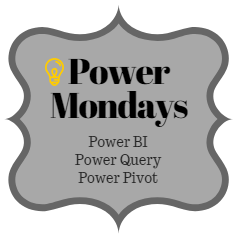Welcome to Power Mondays. Every Monday, learn all about Power BI, Power Query & Power Pivot in full length examples, videos or tips. In the first installment, let’s take a look at something we all can related to – Money.

We all know that Power BI is good for creating awesome visual experiences. Today let me share another fun way to use Power BI – to build a calculator. Learn how to create nest egg calculator in this Power BI parameter example tutorial.

This is what our output looks like:Ready to learn how to do this in Power BI? Read on then…

## Creating a nest egg calculator with Power BI Parameters – Step by step tutorialWe will use a very interesting and little known feature of Power BI – what-if parameters to build our nest egg calculator.

If you are new to Power BI, check out this excellent tutorial before reading rest of this.

### Step 1: Defining goals for the nest egg calculator

Just as building a large nest egg requires patience and planning, our nest egg calculator too needs some planning. But what planning you say? Just thinking out loud what our calculator should look like and what it should do is enough. So on that note, let’s define some goals:

• We have two kinds of savings – regular (monthly) and one time (already invested)
• The expected rate of return is different for each type of saving
• We want to see how the nest egg will grow over time, for example, next 30 years

Let’s say we want to figure out future value of 3 monthly investments and an existing investment. Each has their own expected rate of return.

We need to calculate value of investment at the end of year for next 30 years. ie Future value of our investment.

### Step 2: Set up Power BI parameters to capture inputs

As each of our inputs can change, we need something that let’s us toy with the inputs. Guess what? We will use Parameters. This feature of Power BI let’s you add a what-if parameter to your workbook.

When you add a what-if parameter, Power BI does two things:

• Creates a table with all possible parameter values
• Creates a harvester measure that tells you which value is selected by user

To insert a parameter: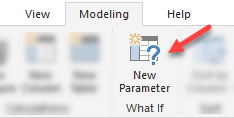Open blank Power BI workbook and using what-if parameter button in the modeling tab, insert a parameter, as below.

#### Power BI Parameter Example – Demo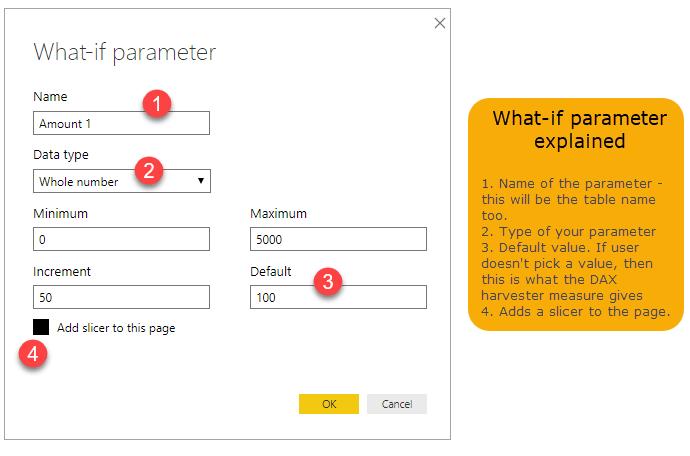Now, repeat this step for 7 more times, so that we end up with 8 parameter tables, as described below.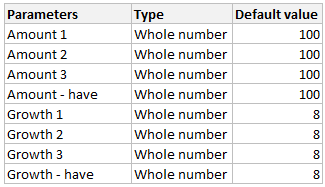At the end of this step, we will have 8 tables and 8 measures.

Lay out the parameter slicers like this on the canvas:

Note: You need to enable slider for the slicers.### Step 3: Create a table for forecastWe want to forecast the future value of investments for next 30 years. That means, we need to know the future value for each of those 30 years. If only, we had a table with numbers 0 to 30, then we can write some sort of DAX formula to calculate the FV.

To start off, let’s generate a table with numbers 0 to 30 (31 rows). You can do this in either Power Pivot (using GENERATESERIES() DAX formula) or in Power Query using the query ={0..30}

Let’s do this in Power Query. To create the forecast table in PQ:

1. Go to home > get data > blank query
2. When a blank query is created in PQ, in the formula bar type ={0..30} and press enter
3. PQ will create a series of 31 numbers (starting from 0 and ending at 30) as a list
4. Convert this list to a table using List tools > Transform ribbon.
5. Add any other columns (derived) if you want.
6. Name this query as Projection and load it to Power BI.
If you are new to Power Query, check out this beginner how to to understand how it works.

### Step 4: Calculate forecast values

Now that our parameters and forecast table are ready, we can calculate future values of each investment. If you have this data in Excel, you can use FV() function to calculate the value. Unfortunately, Power Pivot doesn’t have FV() DAX formula. So how?

Simple, we can write the actual algebra.

The equation for future value of P payment for n periods at r interest rate is:

FV = P*(((1+r)^n – 1) / r)

For example, for [Amount 1 Value] of \$100 invested at [Growth 1 Value] for 5 years would be:

=[Amount 1 Value] * (((1+[Growth 1 Value])^5 – 1)/[Growth 1 Value])

But wait, we are investing monthly…

As we are investing monthly instead of yearly, we need to to change r & n to r/12 and n*12.

So the final formula for future value after 5 years will be:

=[Amount 1 Value] * (((1+[Growth 1 Value]/12)^(5*12) – 1)/([Growth 1 Value]/12))

Replacing the division with DIVIDE() DAX formula, we get:

= [Amount 1 Value] * DIVIDE(((1+[Growth 1 Value]/12)^(5*12)-1),([Growth 1 Value]/12),1)

Calculating for all years

The above DAX formula works only for 5th year. How to calculate for any year?

Simple, we create a measure called as [selected year] which when used in a visual (like chart or table) will return different years. Something like =MAX(Projection[year]) should do.

Replacing 5 with [selected year], we get:

Amount 1 FV := [Amount 1 Value] * DIVIDE(((1+[Growth Pct 1 Value]/12)^([selected year]*12)-1),([Growth Pct 1 Value]/12),1)

Create 2 more such measures for Amount 2 FV and Amount 3 FV.

Calculating Future Value of [Amount have]:

In case of starting amount (existing investments), we can use compound interest logic to calculate future value.

The future value of amount P invested at r interest over n periods is given by this formula:

=P*(1+r)^n

Here is the measure for same:

Already have FV := [Already have Value]*(1+’Growth Pct (have)'[Growth Pct (have) Value])^[selected year]*1000

Remember, already have value is entered in \$000s, so we must multiply the result with 1000.

### Step 5: Visualize the result

And now comes the best part. We visualize all the yummy results calculated by our measures.

Start by inserting a stacked area chart. This is perfect for our calculator.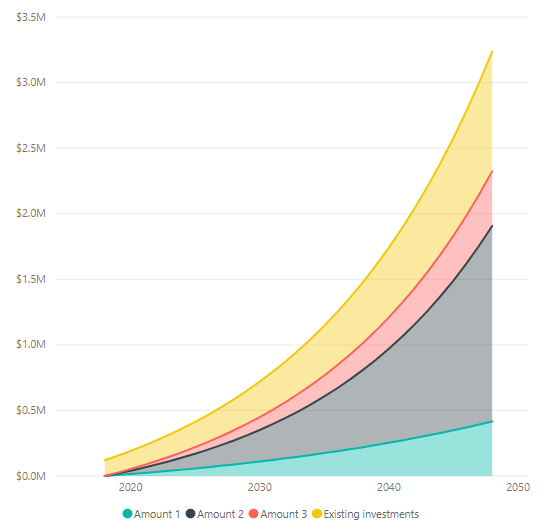When you put years on X axis of this visual, Power BI (thru Power Pivot) calculates the future value of all 4 investments for each year and shows the output as a stacked area chart. Cool no?

Now as you play with the sliders, the future amounts change. Go ahead and find out how much your nest egg will be worth. And then start working towards it.

## See all of it in action – Live retirement savings calculator

Want to play with this but not near Power BI? Just use the embedded Power BI visual below to play and find out how much your nest egg will be worth.

Note: This is made with July 2018 release of PBI, but should work in any recent version. If you notice anything funny, drop a comment so I can help.

### Want more Power? Check out these amazing examples

If you like Power BI, please check out these examples to see other creative ways to use it.

Excited about Power Mondays? Tell me what you want to see more?

I am super excited about Power Mondays. Every Monday, you will see the mighty magic of various Power tools. If you think I should talk about a certain business problem or concept, please post your suggestion in the comments. I will research and write about it on the blog in a subsequent Power Monday episode.

### Get FREE Excel + Power BI Tips

Simple, fun and useful emails, once per week.

Learn & be awesome.

### Welcome to Chandoo.org

Thank you so much for visiting. My aim is to make you awesome in Excel & Power BI. I do this by sharing videos, tips, examples and downloads on this website. There are more than 1,000 pages with all things Excel, Power BI, Dashboards & VBA here. Go ahead and spend few minutes to be AWESOME.

Read my storyFREE Excel tips book

Chandoo is an awesome teacher
5/5

– Jason

Still on fence about Power BI? In this getting started guide, learn what is Power BI, how to get it and how to create your first report from scratch.

## Related Tips

### 6 Responses to “Nest Egg Calculator using Power BI”

1.Sandeep Kothari says:

Wow! What a Powerful article!

2.ravi says:

Hello Chandoo Sir

your file does not work with Excel 2016.
how can I try my hands on this powerful nest egg file ?

thanks

Ravi Santwani

•Chandoo says:

@Ravi... this is a Power BI workbook. You need Power BI Desktop to view it. See the below tutorial to understand what Power BI is:

https://chandoo.org/wp/introduction-to-power-bi/

3.Himanshu Patidar says:

As always, superb article Chandoo... 🙂

Just one minor issue:
While following your steps and replicating this calculator in PowerBI, I found that the Growth Pct Parameters should be set as "Decimal number" not "Whole Number"
OR
we have to make corresponding adjustments in the Forecast formulas (i.e. divide by 100) to get accurate results.

•Chandoo says:

You are right. I used whole number but modified the auto created harvester measure with /100 at end. Sorry I did not mention it in the tutorial.

4.FrankT says:

[Growth Pct 1 Value]/12
the monthly rate has to be
(1+[Growth Pct 1 Value])^(1/12)-1

It's a slight difference but in 30 years the future value will be \$100k less.

### Get FREE Excel & Power-BI Newsletter

One email per week with Excel and Power BI goodness. Join 100,000+ others and get it free.